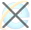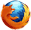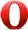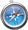HTML output name 属性

HTML <output> name 属性HTML <output> 标签

实例

<form oninput="x.value=parseInt(a.value)+parseInt(b.value)">0
<input type="range" id="a" value="50">100
+<input type="number" id="b" value="50">
=<output name="x" for="a b"></output>
</form>

浏览器支持Firefox、Opera、Chrome 和 Safari 浏览器都支持 name 属性。

定义和用法

name 属性规定 <output> 元素的名称。

name 属性用于在表单提交后引用表单数据，或者用于在 JavaScript 中引用元素。

HTML 4.01 与 HTML5之间的差异

<output> 标签是 HTML5 中的新标签。

语法

<output name="name">

属性值

name规定 <output> 元素的名称。HTML <output> 标签